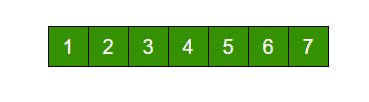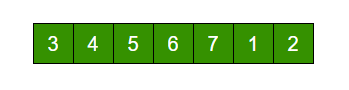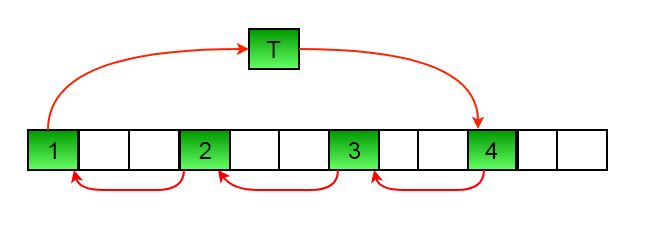Open in App
Not now

# C Program for Program for array rotation

• Last Updated : 19 Jan, 2023

Write a function rotate(arr[], d, n) that rotates arr[] of size n by d elements.Rotation of the above array by 2 will make arrayMethod 1 (Rotate one by one):

```leftRotate(arr[], d, n)
start
For i = 0 to i < d
Left rotate all elements of arr[] by one
end```

To rotate by one, store arr in a temporary variable temp, move arr to arr, arr to arr …and finally temp to arr[n-1]
Let us take the same example arr[] = [1, 2, 3, 4, 5, 6, 7], d = 2
Rotate arr[] by one 2 times
We get [2, 3, 4, 5, 6, 7, 1] after first rotation and [ 3, 4, 5, 6, 7, 1, 2] after second rotation.

Method 2 (A Juggling Algorithm) :
This is an extension of method 2. Instead of moving one by one, divide the array in different sets
where number of sets is equal to GCD of n and d and move the elements within sets.
If GCD is 1 as is for the above example array (n = 7 and d =2), then elements will be moved within one set only, we just start with temp = arr and keep moving arr[I+d] to arr[I] and finally store temp at the right place.
Here is an example for n =12 and d = 3. GCD is 3 and

```Let arr[] be {1, 2, 3, 4, 5, 6, 7, 8, 9, 10, 11, 12}
a) Elements are first moved in first set – (See below
diagram for this movement)``````      arr[] after this step --> {4 2 3 7 5 6 10 8 9 1 11 12}

b)    Then in second set.
arr[] after this step --> {4 5 3 7 8 6 10 11 9 1 2 12}

c)    Finally in third set.
arr[] after this step --> {4 5 6 7 8 9 10 11 12 1 2 3}```

Below is the implementation of the above approach :

## C

 `// C program to rotate an array by``// d elements``#include ` `/* function to print an array */``void` `printArray(``int` `arr[], ``int` `size);` `/*Function to get gcd of a and b*/``int` `gcd(``int` `a, ``int` `b);` `/*Function to left rotate arr[] of size n by d*/``void` `leftRotate(``int` `arr[], ``int` `d, ``int` `n)``{``    ``int` `i, j, k, temp;``    ``/* To handle if d >= n */``    ``d = d % n;``    ``int` `g_c_d = gcd(d, n);``    ``for` `(i = 0; i < g_c_d; i++) {``        ``/* move i-th values of blocks */``        ``temp = arr[i];``        ``j = i;``        ``while` `(1) {``            ``k = j + d;``            ``if` `(k >= n)``                ``k = k - n;``            ``if` `(k == i)``                ``break``;``            ``arr[j] = arr[k];``            ``j = k;``        ``}``        ``arr[j] = temp;``    ``}``}` `/*UTILITY FUNCTIONS*/``/* function to print an array */``void` `printArray(``int` `arr[], ``int` `n)``{``    ``int` `i;``    ``for` `(i = 0; i < n; i++)``        ``printf``(``"%d "``, arr[i]);``}` `/*Function to get gcd of a and b*/``int` `gcd(``int` `a, ``int` `b)``{``    ``if` `(b == 0)``        ``return` `a;``    ``else``        ``return` `gcd(b, a % b);``}` `/* Driver program to test above functions */``int` `main()``{``    ``int` `arr[] = { 1, 2, 3, 4, 5, 6, 7 };``    ``leftRotate(arr, 2, 7);``    ``printArray(arr, 7);``    ``getchar``();``    ``return` `0;``}`

Output :

`3 4 5 6 7 1 2 `

Time complexity : O(n)
Auxiliary Space : O(1)

My Personal Notes arrow_drop_up